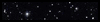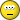2108 Posts
  Prev «  48 , 49 , 50 , 51 , 52  Next »   |

## Subject: Free microbadge coupons {for rolling 1899}D2000 = (1250) = 1250
• [+] Dice rolls
• [+]
• 1478095. DraedGhawl
• D2000 =
• (1250) =
• 1250
• Sun Jun 23, 2019 11:45 pm
1d2000 = (486) = 486
• [+] Dice rolls
• [+]
• 1478106. ghostpants
• 1d2000 =
• (486) =
• 486
• Mon Jun 24, 2019 1:26 am
d2000 = (1695) = 1695
• [+] Dice rolls
• [+]
• 1478141. CrazyCatman
• d2000 =
• (1695) =
• 1695
• Mon Jun 24, 2019 7:05 am
d2000 = (1877) = 1877
• [+] Dice rolls
• [+]
• 1478249. shelflife3000
• d2000 =
• (1877) =
• 1877
• Mon Jun 24, 2019 3:23 pm
1d2000 = (1118) = 1118
• [+] Dice rolls
• [+]
• 1478345. yaverot
• 1d2000 =
• (1118) =
• 1118
• Mon Jun 24, 2019 7:11 pm
d2000 = (1972) = 1972
• [+] Dice rolls
• [+]
• 1478412. bulldog93
• d2000 =
• (1972) =
• 1972
• Mon Jun 24, 2019 9:50 pm
1d2000 = (1001) = 1001
• [+] Dice rolls
• [+]
• 1478423. ghostpants
• 1d2000 =
• (1001) =
• 1001
• Mon Jun 24, 2019 10:36 pm
d2000 = (1151) = 1151
• [+] Dice rolls
• [+]
• 1478430. DraedGhawl
• d2000 =
• (1151) =
• 1151
• Mon Jun 24, 2019 11:03 pm
Some days you just gotta get d2000 = (435) = 435donuts in your mouth.
• [+] Dice rolls
• [+]
• 1478450. USP45
• d2000 =
• (435) =
• 435
• Some days you just gotta get
• Tue Jun 25, 2019 12:27 am
☆ ✧ ☆ ✧ ☆
United States
Minneapolis
Minnesota
Looking at these stars suddenly dwarfed my own troubles and all the gravities of terrestrial life. I thought of their unfathomable distance, and the slow inevitable drift of their movements out of the unknown past into the unknown future. H.G. WellsHumankind has not woven the web of life. We are but one thread within it. Whatever we do to the web, we do to ourselves. All things are bound together. All things connect. Chief Seattle
1d2000 = (680) = 680
• [+] Dice rolls
• [+]
• 1478470. frumpish
• 1d2000 =
• (680) =
• 680
• Tue Jun 25, 2019 3:12 am
USP45 wrote:
USP45 previously rolled d2000 = (435) = 435 (Some days you just gotta get) donuts in your mouth.
Are you a cop?d2000 = (1455) = 1455
• [+] Dice rolls
• [+]
• 1478530. CrazyCatman
• d2000 =
• (1455) =
• 1455
• Tue Jun 25, 2019 10:26 am
1d2000 = (1468) = 1468
• [+] Dice rolls
• [+]
• 1478609. yaverot
• 1d2000 =
• (1468) =
• 1468
• Tue Jun 25, 2019 2:44 pm
d2000 = (1843) = 1843
• [+] Dice rolls
• [+]
• 1478616. shelflife3000
• d2000 =
• (1843) =
• 1843
• Tue Jun 25, 2019 3:07 pm
1d2000 = (1622) = 1622
• [+] Dice rolls
• [+]
• 1478625. Narl
• 1d2000 =
• (1622) =
• 1622
• Tue Jun 25, 2019 3:17 pm
I would love to have d2000 = (1857) = 1857 dogs running around my backyard.
• [+] Dice rolls
• [+]
• 1478789. USP45
• d2000 =
• (1857) =
• 1857
• I would love to have
• Tue Jun 25, 2019 10:20 pm
D2000 = (1955) = 1955
• [+] Dice rolls
• [+]
• 1478871. DraedGhawl
• D2000 =
• (1955) =
• 1955
• Wed Jun 26, 2019 12:39 am
1d2000 = (1443) = 1443
• [+] Dice rolls
• [+]
• 1478885. ghostpants
• 1d2000 =
• (1443) =
• 1443
• Wed Jun 26, 2019 1:08 am
d2000 = (685) = 685
• [+] Dice rolls
• [+]
• 1479016. CrazyCatman
• d2000 =
• (685) =
• 685
• Wed Jun 26, 2019 11:30 am
1d2000 = (1599) = 1599
• [+] Dice rolls
• [+]
• 1479037. Narl
• 1d2000 =
• (1599) =
• 1599
• Wed Jun 26, 2019 12:07 pm
d2000 = (1532) = 1532
• [+] Dice rolls
• [+]
• 1479147. bulldog93
• d2000 =
• (1532) =
• 1532
• Wed Jun 26, 2019 4:50 pm
Only d2000 = (1137) = 1137 more minutes left in this swim meet.
• [+] Dice rolls
• [+]
• 1479347. USP45
• d2000 =
• (1137) =
• 1137
• Only
• Thu Jun 27, 2019 12:06 am
d2000 = (1583) = 1583
• [+] Dice rolls
• [+]
• 1479366. shelflife3000
• d2000 =
• (1583) =
• 1583
• Thu Jun 27, 2019 2:14 am
1d2000 = (888) = 888
• [+] Dice rolls
• [+]
• 1479387. yaverot
• 1d2000 =
• (888) =
• 888
• Thu Jun 27, 2019 2:50 am
d2000 = (573) = 573
• [+] Dice rolls
• [+]
• 1479438. CrazyCatman
• d2000 =
• (573) =
• 573
• Thu Jun 27, 2019 9:12 am
d2000 = (520) = 520
• [+] Dice rolls
• [+]
• 1479447. DraedGhawl
• d2000 =
• (520) =
• 520
• Thu Jun 27, 2019 10:53 am
  Prev «  48 , 49 , 50 , 51 , 52  Next »   |